# 【MTSP】基于matlab遗传算法求解多旅行商问题【含Matlab源码 1339期】

## 二、遗传算法简介

1 引言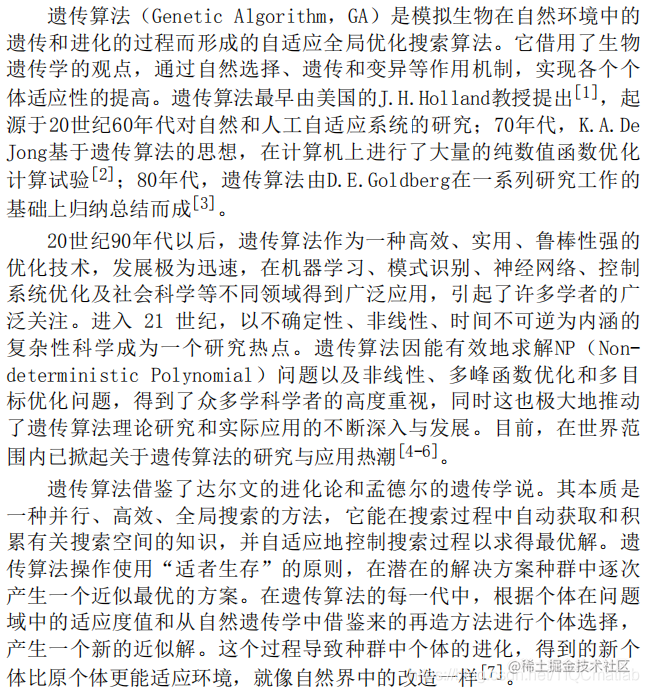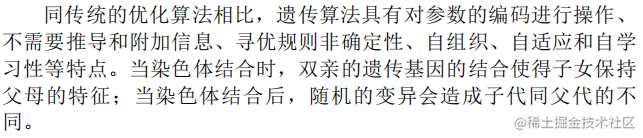2 遗传算法理论 2.1 遗传算法的生物学基础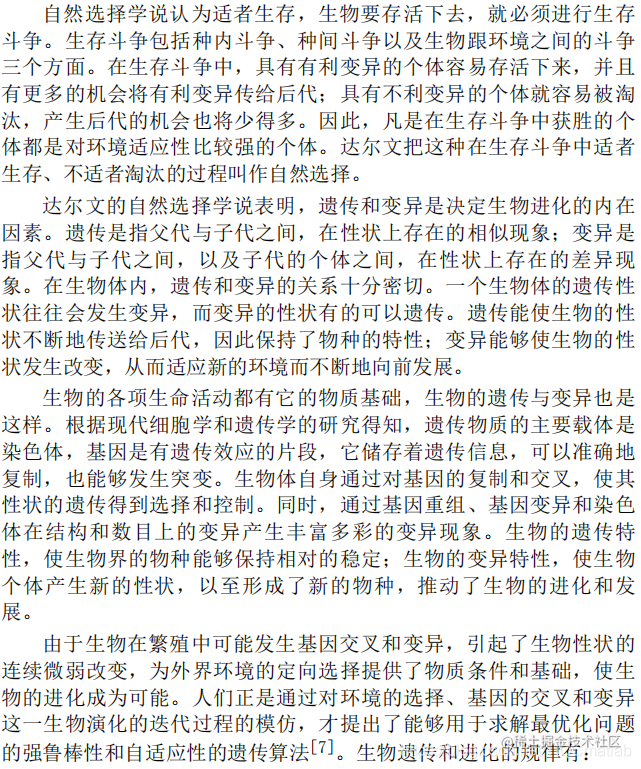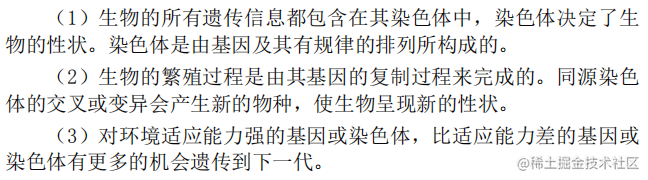2.2 遗传算法的理论基础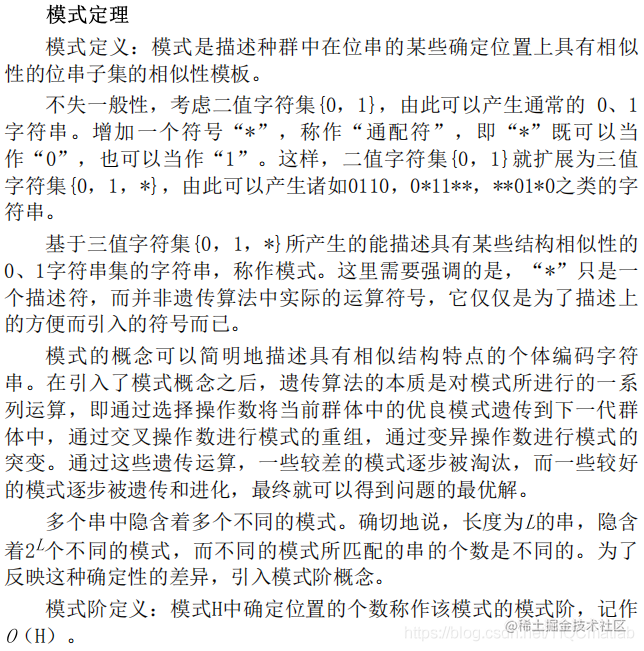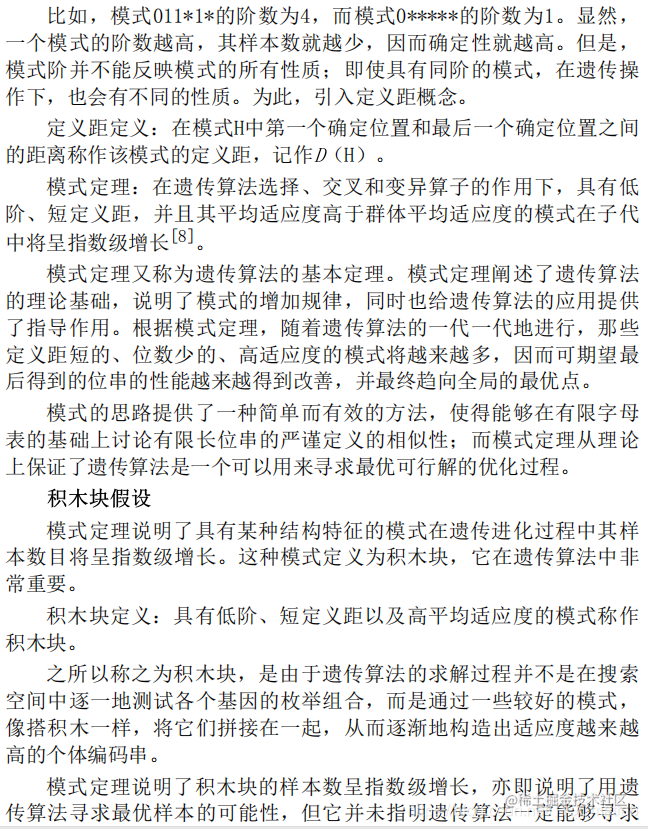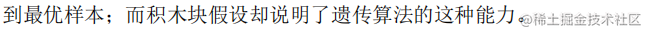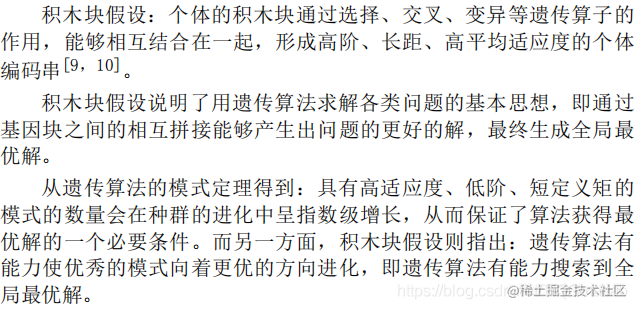2.3 遗传算法的基本概念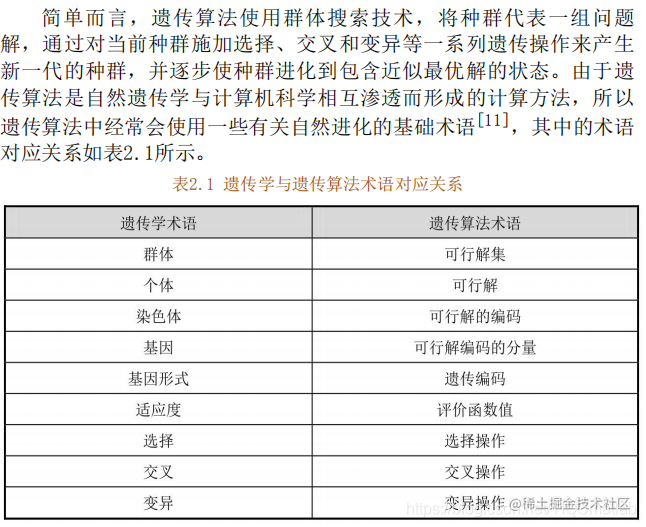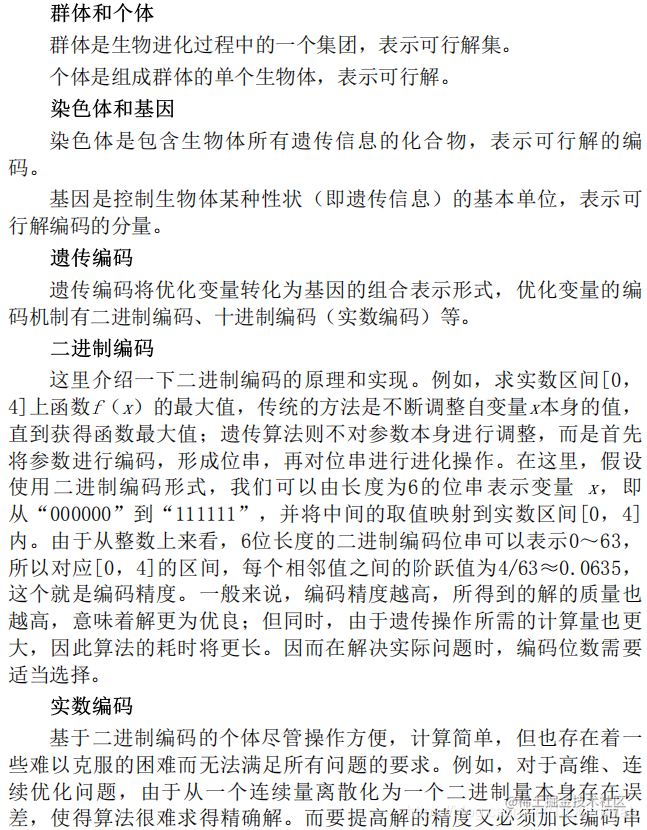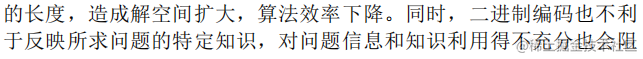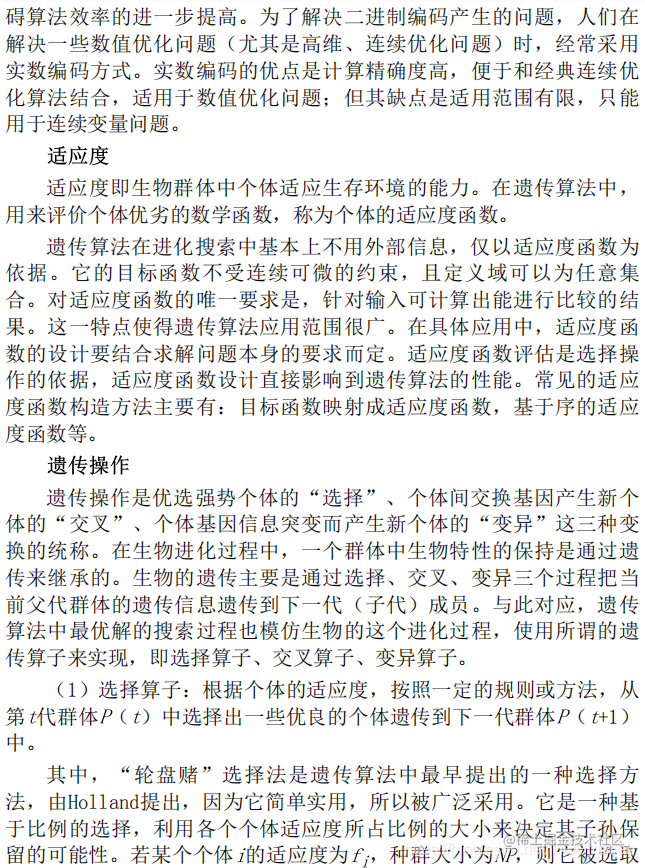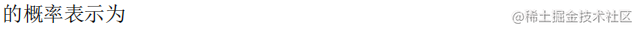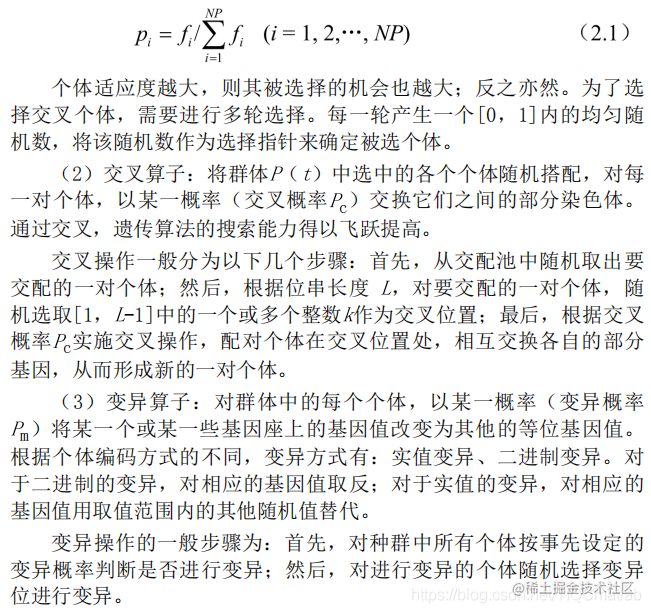2.4 标准的遗传算法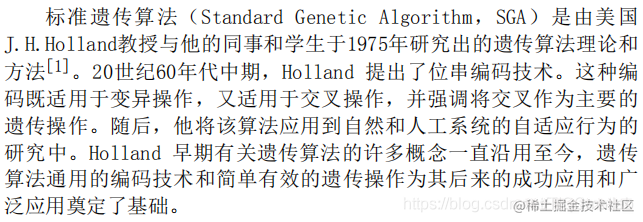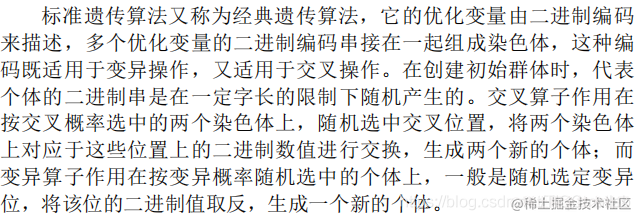2.5 遗传算法的特点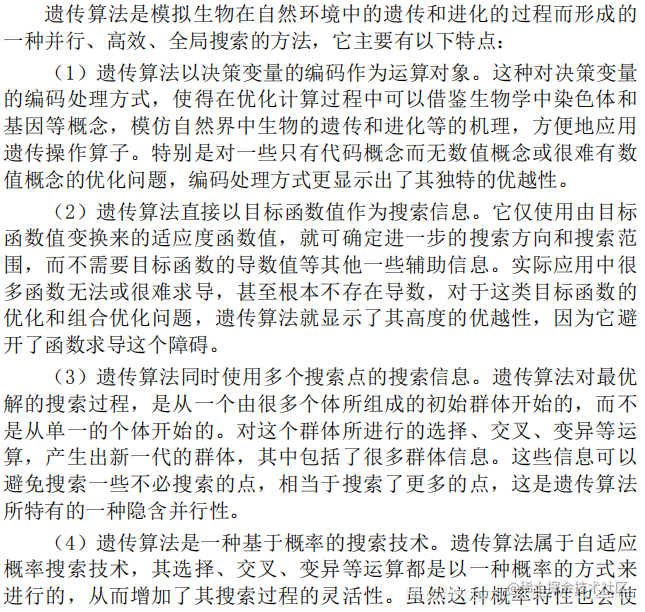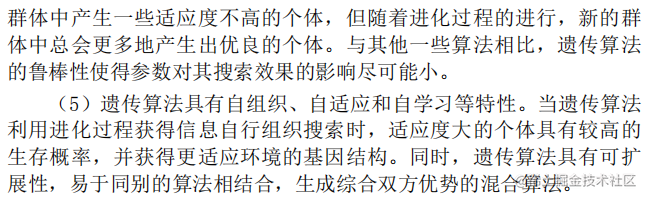2.6 遗传算法的改进方向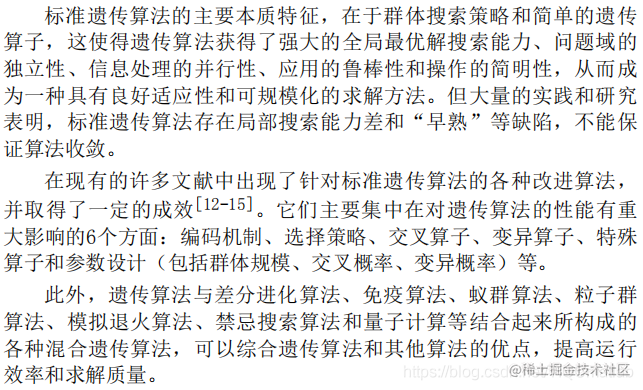3 遗传算法流程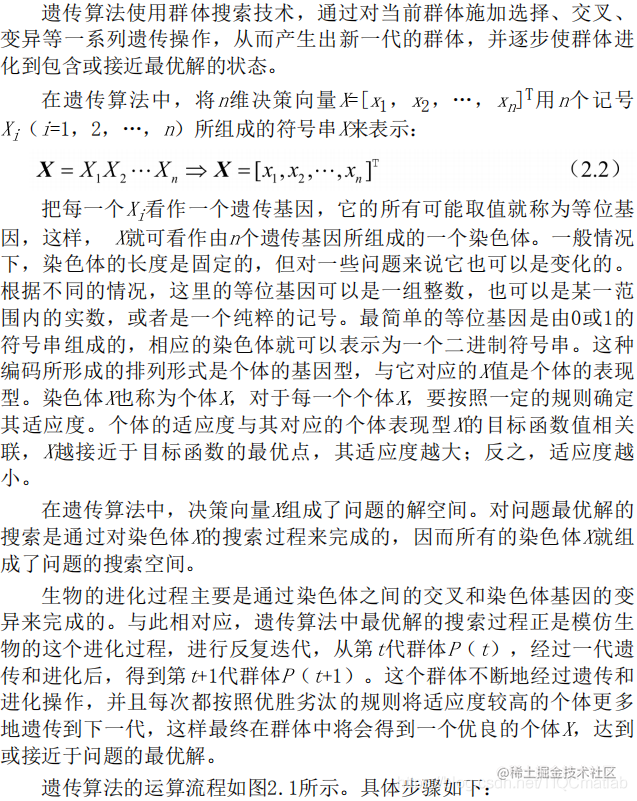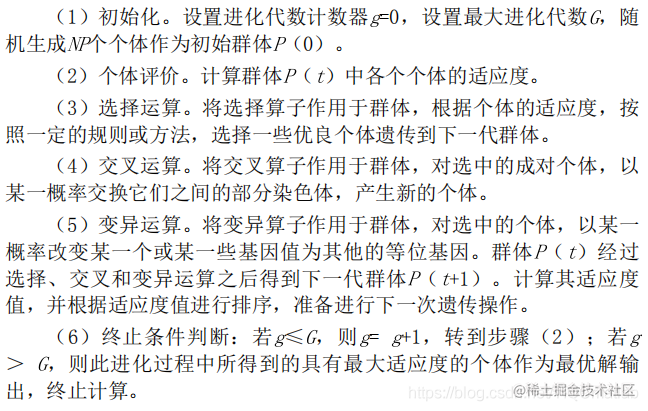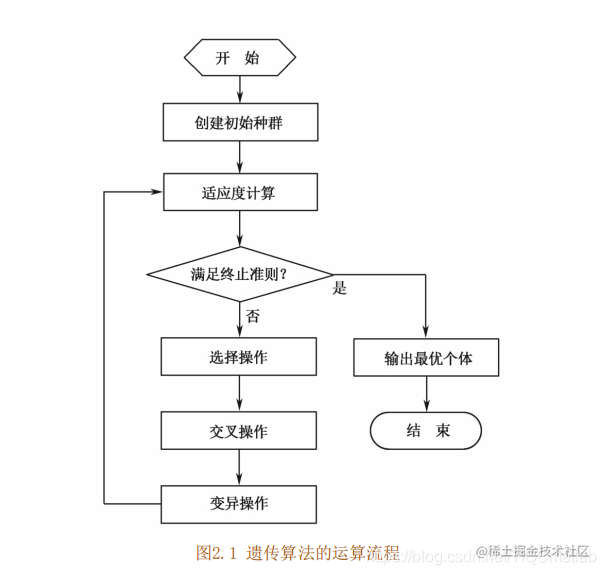4 关键参数说明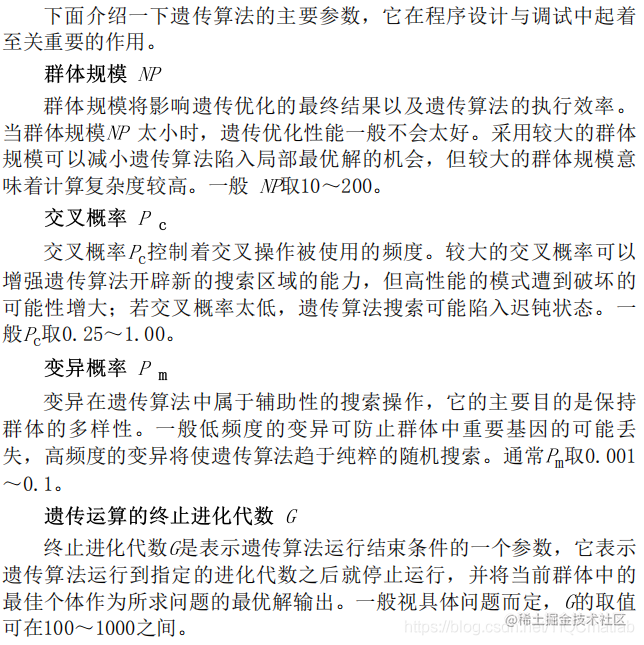## 三、部分源代码

``````function [min_dist,best_tour,generation] = mdmtspv_ga(xy,max_salesmen,depots,CostType,min_tour,pop_size,num_iter,show_prog,show_res,dmat)
% MDMTSPV_GA Multiple Depots Multiple Traveling Salesmen Problem (M-TSP)
% with Variable number of salesmen using Genetic Algorithm (GA)
%   Finds a (near) optimal solution to a variation of the M-TSP (that has a
%   variable number of salesmen) by setting up a GA to search for the
%   shortest route (least distance needed for the salesmen to travel to
%   each city exactly once and return to their starting locations). The
%   salesmen originate from a set of fixed locations, called depots.
%   This algorithm is based on Joseph Kirk's MTSPV_GA, but adds the
%   following functionality:
%     1. Depots at which each salesman originates and ends its tour.
%     2. Two possible cost functions, that allow to find minimum sum of all
%        tour lengths (as in the original version) and to find the minimum
%        longest tour. The latter problem is sometimes called MinMaxMDMTSP.
%
% Summary:
%     1. Each salesman travels to a unique set of cities and completes the
%        route by returning to the depot he started from
%     2. Each city is visited by exactly one salesman
%
% Input:
%     XY (float) is an Nx2 matrix of city locations, where N is the number of cities
%     max_salesmen (scalar integer) is the maximum number of salesmen
%     depots (float)  ia an Mx2 matrix of the depots used by salesmen, M=max_salesmen
%     CostType (integer) defines which cost we use. If 1 - sum of all route lengths, if 2 - maximum route length%     MIN_TOUR (scalar integer) is the minimum tour length for any of the salesmen
%     POP_SIZE (scalar integer) is the size of the population (should be divisible by 16)
%     NUM_ITER (scalar integer) is the number of desired iterations for the
%       algorithm to run after a new best solution is found. Don't worry the
%       algorithm will always stop.
%     SHOW_PROG (scalar logical) shows the GA progress if true
%     SHOW_RES (scalar logical) shows the GA results if true
%     DMAT (float) is an NxN matrix of point to point distances or costs

%
% Output:
%     MIN_DIST (scalar float) is the best cost found by the algorithm
%     BEST_TOUR (matrix integer) is an MxL matrix, each row is an agent tour
%     Generation (scalar integer) is the number of generations required by
%       the algorithm to find the solution

%
% Route/Breakpoint Details:
%     The algorithm uses a data structure in which RTE lists the cities in
%     a route and BRKS lists break points that divide RTE  between agents.
%     If there are 10 cities and 3 salesmen, a possible route/break
%     combination might be: rte = [5 6 9 1 4 2 8 10 3 7], brks = [3 7]
%     Taken together, these represent the solution [5 6 9][1 4 2 8][10 3 7],
%     which designates the routes for the 3 salesmen as follows:
%         . Salesman 1 travels from city 5 to 6 to 9 and back to 5
%         . Salesman 2 travels from city 1 to 4 to 2 to 8 and back to 1
%         . Salesman 3 travels from city 10 to 3 to 7 and back to 10
%     Note that the salesman's depot will be taken into accout, so the
%     complete routes returned by the algorithm will be:
%         For agent 1: [1 5 6 9 1] - from depot 1 along the route and back
%         For agent 2: [2 1 4 2 8 2] - from depot 2 along the route and back
%         For agent 3: [3 10 3 7 3] - from depot 3 along the rout and back
%
% 2D Example:
%     n = 35;
%     xy = 10*rand(n,2);
%     max_salesmen = 5;
%     depots = 10*rand(max_salesmen,2);
%     CostType=1; %- total length, use 2 to minimize the longest tour
%     min_tour = 3;
%     pop_size = 80;
%     num_iter = 1e3;
%     a = meshgrid(1:n);
%     dmat = reshape(sqrt(sum((xy(a,:)-xy(a',:)).^2,2)),n,n);
%     [min_dist,best_tour,generation] = mdmtspv_ga(xy,max_salesmen,depots,CostType,min_tour,pop_size,num_iter,1,1,dmat)
%
% 3D Example:
%     n = 35;
%     xy = 10*rand(n,3);
%     max_salesmen = 5;
%     depots = 10*rand(max_salesmen,3);
%     CostType=1; %- total length, use 2 to minimize the longest tour
%     min_tour = 3;
%     pop_size = 80;
%     num_iter = 1e3;
%     a = meshgrid(1:n);
%     dmat = reshape(sqrt(sum((xy(a,:)-xy(a',:)).^2,2)),n,n);
%     [min_dist,best_tour,generation] = mdmtspv_ga(xy,max_salesmen,depots,CostType,min_tour,pop_size,num_iter,1,1,dmat)
%
% See also: mtsp_ga, mtspf_ga, mtspo_ga, mtspof_ga, mtspofs_ga, distmat

% Process Inputs and Initialize Defaults
nargs = 10;
for k = nargin:nargs-1
switch k
case 0
xy = 10*rand(40,2);
case 1
max_salesmen=10;
case 2
depots = 10*rand(max_salesmen,2);
case 3
CostType = 2;
case 4
min_tour = 1;
case 5
pop_size = 80;
case 6
num_iter = 1e3;
case 7
show_prog = 1;
case 8
show_res = 1;
case 9
N = size(xy,1);
a = meshgrid(1:N);
dmat = reshape(sqrt(sum((xy(a,:)-xy(a',:)).^2,2)),N,N);
otherwise
end
end

Epsilon=1e-10;

% Distances to Depots
%Assumes that each salesman is located at a different depot and there are
%enough depots
[NumOfCities,Dimensions]=size(xy);
for i=1:max_salesmen
for j=1:NumOfCities
D0(i,j)=norm(depots(i,:)-xy(j,:));
end
end

% Verify Inputs
[N,dims] = size(xy);
[nr,nc] = size(dmat);
if N ~= nr || N ~= nc
error('Invalid XY or DMAT inputs!')
end
n = N;

% Sanity Checks
min_tour = max(1,min(n,round(real(min_tour(1)))));
pop_size = max(8,8*ceil(pop_size(1)/8));
num_iter = max(1,round(real(num_iter(1))));
show_prog = logical(show_prog(1));
show_res = logical(show_res(1));

% Initialize the Populations
pop_rte = zeros(pop_size,n);	% population of routes
pop_brk = cell(pop_size,1);     % population of breaks
for k = 1:pop_size
pop_rte(k,:) = randperm(n);
pop_brk{k} = randbreak(max_salesmen,n,min_tour);
end

% Select the Colors for the Plotted Routes
%clr = hsv(ceil(n/min_tour));
clr = hsv(max_salesmen);

% Run the GA
global_min = Inf;
total_dist = zeros(1,pop_size);
dist_history = zeros(1,num_iter);
tmp_pop_rte = zeros(8,n);
tmp_pop_brk = cell(8,1);
new_pop_rte = zeros(pop_size,n);
new_pop_brk = cell(pop_size,1);
if show_prog
pfig = figure('Name','MTSPV_GA | Current Best Solution','Numbertitle','off');
end
iter=0;
iter2go=0;
while iter2go < num_iter
iter2go=iter2go+1;
iter=iter+1;
% Evaluate Each Population Member (Calculate Total Distance)
for p = 1:pop_size
d = [];
p_rte = pop_rte(p,:);
p_brk = pop_brk{p};
salesmen = length(p_brk)+1;
rng=CalcRange(p_brk,n);
for sa = 1:salesmen
if rng(sa,1)<=rng(sa,2)
Tour=[sa p_rte(rng(sa,1):rng(sa,2)) sa];
indices=length(Tour)-1;
d(sa)=CalcTourLength(Tour,dmat,D0,indices);
else
Tour=[sa sa];
d(sa)=0;
end
end
if CostType==1
total_dist(p) = sum(d);
elseif CostType==2
total_dist(p) = max(d)+Epsilon*sum(d);
end
end

% Find the Best Route in the Population
[min_dist,index] = min(total_dist);
dist_history(iter) = min_dist;
if min_dist < global_min
iter2go=0;
generation=iter;
global_min = min_dist;
opt_rte = pop_rte(index,:);
opt_brk = pop_brk{index};
salesmen = length(opt_brk)+1;
rng=CalcRange(opt_brk,n);
if show_prog
% Plot the Best Route
figure(pfig);
clf
for s = 1:salesmen
if dims==2
plot(depots(s,1),depots(s,2),'s','Color',clr(s,:));
else
plot3(depots(s,1),depots(s,2),depots(s,3),'s','Color',clr(s,:));
end
if rng(s,1)<=rng(s,2)
rte = opt_rte([rng(s,1):rng(s,2)]);
hold on;
if ~isempty(rte) && dims == 2
plot(xy(rte,1),xy(rte,2),'.-','Color',clr(s,:));
plot([depots(s,1),xy(rte(1),1)],[depots(s,2),xy(rte(1),2)],'Color',clr(s,:));
plot([depots(s,1),xy(rte(end),1)],[depots(s,2),xy(rte(end),2)],'Color',clr(s,:));
elseif ~isempty(rte) && dims == 3
plot3(xy(rte,1),xy(rte,2),xy(rte,3),'.-','Color',clr(s,:));
plot3([depots(s,1),xy(rte(1),1)],[depots(s,2),xy(rte(1),2)],[depots(s,3),xy(rte(1),3)],'Color',clr(s,:));
plot3([depots(s,1),xy(rte(end),1)],[depots(s,2),xy(rte(end),2)],[depots(s,3),xy(rte(end),3)],'Color',clr(s,:));
end
end
title(sprintf(['Total Distance = %1.4f, Salesmen = %d, ' ...
'Iteration = %d'],min_dist,salesmen,iter));
hold on
end
pause(0.02)
hold off
end
end

## 四、运行结果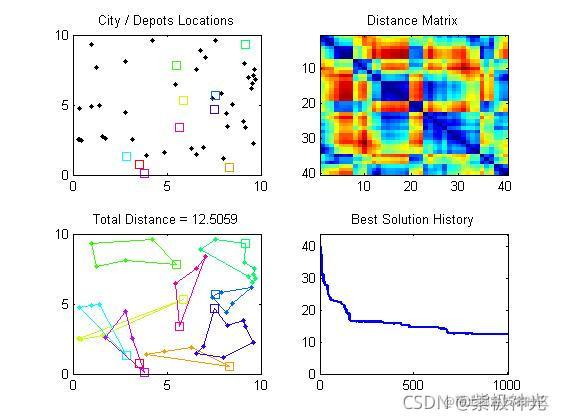## 五、matlab版本及参考文献

1 matlab版本 2014a

2 参考文献  包子阳,余继周,杨杉.智能优化算法及其MATLAB实例（第2版）[M].电子工业出版社，2016. 张岩,吴水根.MATLAB优化算法源代码[M].清华大学出版社，2017.